# Binet formula without floating point

As is well known, Fibonacci numbers are an integer sequence, the first two terms of which are equal to one, and each subsequent one is equal to the sum of the two previous ones. Over the 500 years that have passed since the introduction of this sequence into mathematical use, it has been thoroughly studied. Many interesting formulas involving Fibonacci numbers have been discovered. But one of the “enduring” educational tasks is the calculation of Fibonacci numbers. There are many ways to do this: from direct recursion based on the formula: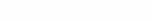to the matrix method described, for example, in the book by D. Knuth . Most of these approaches (except Knuth’s matrix method) are based on the recurrent properties of the Fibonacci sequence and make it possible to calculate the value of Fn in O(n) time at best. Knuth’s matrix method (using matrix exponentiation) calculates the Fibonacci number in logarithmic time .

Binet’s formula (also known to De Moivre) stands apart in this series of algorithms, which has the form: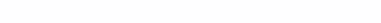This formula seems attractive at first glance, but it contains an irrational number, which in computer calculations we are forced to represent in the form of a floating point number (that is, replace an infinite non-periodic fraction with a finite one).

This means that the calculations will not be accurate; they introduce a constraint error. I once came across a post  which used Binet’s formula to calculate a very large Fibonacci number, but the implementation assumed the use of ultra-high-capacity floating arithmetic (so that the desired number fits completely into the mantissa).

We will go in a completely different way!

First, consider a set of numbers of the form: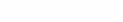for integers a and b. It is easy to see that this set is algebraically closed under the usual addition and multiplication operations: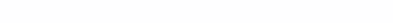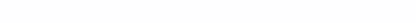Moreover, multiplication and addition will be commutative, which is also easily verified directly. In addition, zero and one fit well into the set under consideration: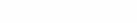The subtraction of such numbers is also quite naturally implemented:You can also define division (of course, only in the case when the divisor is different from zero). The result of division can be defined as the root of the equation: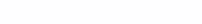Let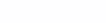Then the previous equality is equivalent to the following: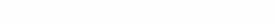Expanding the product on the left side of the last equality, we obtain a system of linear equations for determining the unknowns e and f: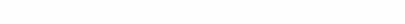From here: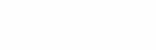The main determinant of this system is: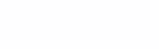Since a and b are integers here, the value of the determinant is always different from zero, which means that the system has a unique solution and division is determined correctly. However, we got carried away. We don’t need division.

We came to the conclusion that the set under consideration with the operations of addition and multiplication forms a ring .

And now – the most important thing! Why do we need a root of five? No one bothers to implement arithmetic on the set of pairs (a, b), in which addition, subtraction and multiplication will be described by formulas: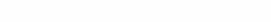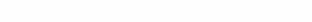Thus, you can “safely forget” about the root of five and implement a direct calculation using the Binet formula. In order for the value to turn out to be an integer (and the root of five to decrease), it is necessary that the numerator of the fraction of the Binet formula be a number of the form: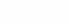which we will identify with the “ordinary” number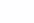Dividing this “ordinary” irrational number by the root of five will give us the desired integer result. Naturally, in reality, division is not required, it is enough to calculate (using the pair arithmetic described above) two binomials: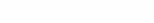andand then do the subtraction. It is not difficult to implement this approach in any programming language. We will do this in Python (the unlimited bit depth of integers in this language attracts).

``````def prod_pairs(a,b):
return (a*b+5*a*b,a*b+a*b)
def sub_pairs(a,b):
return (a-b,a-b)
def pow_pair(a,n):
c=a
for _ in range(n-1):
c=prod_pairs(c,a)
return c
def fib_bine(n):
x1=pow_pair((1,1),n)
x2=pow_pair((1,-1),n)
z=sub_pairs(x1,x2)
return z//(2**n)
``````

Comments are unnecessary – everything is very simple. The question immediately arises, is it possible to speed up this code? Obviously, the “bottleneck” here is the raising of a pair to an integer power. To speed up this operation, there is a standard technique – fast exponentiation (the author also used the same technique ). The idea behind the speedup is that to compute xn the chain x -> x is calculated2 -> x4 ->…->x2k until 2k<=n and then x is calculated in the same way(n-2k).

Now let’s implement a quick exponentiation of a pair to an integer power:

``````def pow_pair(a,n):
if (n==1):
return a
c=copy(a)
k=1
while k*2<=n:
if k<=n:
c=prod_pairs(c,c)
k=k*2
p=n-k
if p>=1:
tmp=pow_pair(a,p)
return prod_pairs(tmp,c)
else:
return c
``````

Using this technique allows you to calculate the Fibonacci numbers in a time close to the logarithmic Binet formula and without using floating point arithmetic. To compare the performance of the proposed method with a method based on simple recursion, the following simple code is written:

``````def fib_ite(n):
c,p=0,1
for _ in range(n):
c,p=c+p,c
return c
``````

And what? Despite the apparent simplicity of the fib_ite code, the fib_bine function performs significantly better. So, on the author’s computer, the four hundred thousandth Fibonacci number is calculated by the described algorithm in about 2 seconds, and by direct iterations – in 27 seconds. The attached figure shows the test results:

The number of the calculated Fibonacci number is plotted along the horizontal axis, and the time in seconds is plotted along the vertical axis.

It turns out that Binet’s formula is quite suitable for fast and accurate calculations of Fibonacci numbers.

Thank you for reading to the end, and also sincere thanks to the authors that I referred to in this note:

one. D. Knut The Art of Computer Programming, v.1, Basic Algorithms. – M: Williams, – 2017. – 720 C.

2. Nth Fibonacci number in O(log N) https://habr.com/en/post/148336/

3. Calculation of the millionth Fibonacci number https://habr.com/ru/company/skillfactory/blog/555914/

4. S.Leng Algebra. M.: Nauka, – 1965. – 431 C.